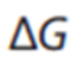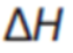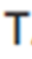# Gibbs Free Energy

How can enthalpy and entropy be used to explain reaction spontaneity?

Learn.

Gibbs free energy is a useful formula we can use to determine whether chemical species which we mix together are going to react with one another in the given conditions. It is important to remember a chemical reaction is trying to achieve greater stability – the following formula can tell us whether a reaction would achieve a more stable configuration:

•is the gibbs free energy (kJ),
• If this is negative the reaction is spontaneous in the given conditions
• If this is zero the reaction is at equilibrium
• If this is positive the reaction is not spontaneous in the given conditions
•Is the change in enthalpy (kJ) – this means the change in the total heat content of the system
• If this value is positive the system is endothermic
• If this value is positive the system is exothermic
•is the temperature of the system in kelvin (K) (be careful a common mistake is to use this in degrees)
• When going from degrees celsius to kelvin, add 273.15
• When going from kelvin to degrees celsius, subtract 273.15
•Is the change in entropy (kJ/K). Entropy is the measure of disorder of a system. So if your room is really messy and chaotic, then it has high entropy. Disorder is more probable then order hence a system tends to become more disorder over time. However, it is important to keep in mind a system can become more ordered.

Entropy is increasing if:

• From (l) → (g)
• From (s) → (l)
• From (s) → (g)
• Fewer moles → more moles

This table summarises the conditions’ effect on Gibbs free energy, however, it is recommended to derive these by looking at the equation to minimise the need to memorise.

Memorize.

Read our post that teaches you how to make memorizing easier here.

Make the lesson stick and get this as a sticker here

Master.

Question 1.

IS the following reaction spontaneous or non spontaneous if the reaction vessel is at 273.1 K, the delta H is 273, delta S is 180 kJ/K?

Question 2.

Reactants are added to a reaction vessel at 244 degrees celsius, the H of products is 88 kJ/mol and the H of reactants is 164 kJ/mol. The delta S of this reaction is 273 kJ/K.

Is the reaction spontaneous or non spontaneous?

Question 3.

Delta S is 3400 J/K and Delta H is 270 kJ.

What temperature, if any, is required for this reaction to be spontaneous?

Question 1.

Delta G = -48885 kJ

Therefore the reaction is spontaneous.

Question 2.

244 -273 = -29K

164 – 88 = 76kJ

Delta G = 7993 kJ

Therefore the reaction is non spontaneous

Question 3.

3400/1000 = 3.4kJ

Delta G is negative ( Therefore, delta G < 0)

0 < 270 – T(3.4)

270 < T(3.4)

79.4 < T

T  > 79.4

Learning Outcomes.

• analyse the differences between entropy and enthalpy
• use modelling to illustrate entropy changes in reactions
• predict entropy changes from balanced chemical reactions to classify as increasing or decreasing
entropy
• explain reaction spontaneity using terminology, including:
• Gibbs free energy
• Enthalpy
• Entropy
• solve problems using standard references and 𝛥𝐺𝑜 = 𝛥𝐻 𝑜 − 𝑇𝛥𝑆𝑜
(Gibbs free energy formula) to
classify reactions as spontaneous or non-spontaneous
• predict the effect of temperature changes on spontaneity